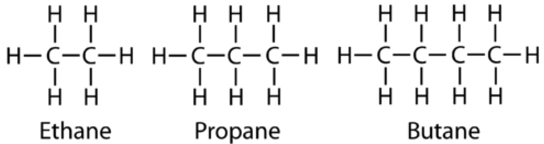# 11.2: Straight-Chain Alkanes

$$\newcommand{\vecs}{\overset { \rightharpoonup} {\mathbf{#1}} }$$ $$\newcommand{\vecd}{\overset{-\!-\!\rightharpoonup}{\vphantom{a}\smash {#1}}}$$$$\newcommand{\id}{\mathrm{id}}$$ $$\newcommand{\Span}{\mathrm{span}}$$ $$\newcommand{\kernel}{\mathrm{null}\,}$$ $$\newcommand{\range}{\mathrm{range}\,}$$ $$\newcommand{\RealPart}{\mathrm{Re}}$$ $$\newcommand{\ImaginaryPart}{\mathrm{Im}}$$ $$\newcommand{\Argument}{\mathrm{Arg}}$$ $$\newcommand{\norm}{\| #1 \|}$$ $$\newcommand{\inner}{\langle #1, #2 \rangle}$$ $$\newcommand{\Span}{\mathrm{span}}$$ $$\newcommand{\id}{\mathrm{id}}$$ $$\newcommand{\Span}{\mathrm{span}}$$ $$\newcommand{\kernel}{\mathrm{null}\,}$$ $$\newcommand{\range}{\mathrm{range}\,}$$ $$\newcommand{\RealPart}{\mathrm{Re}}$$ $$\newcommand{\ImaginaryPart}{\mathrm{Im}}$$ $$\newcommand{\Argument}{\mathrm{Arg}}$$ $$\newcommand{\norm}{\| #1 \|}$$ $$\newcommand{\inner}{\langle #1, #2 \rangle}$$ $$\newcommand{\Span}{\mathrm{span}}$$$$\newcommand{\AA}{\unicode[.8,0]{x212B}}$$

As our country looks at the prospect of oil shortages in the future, we are searching for alternative transportation fuel sources. One very viable possibility is propane gas. Power and acceleration capacities for propane-powered vehicles are comparable to gasoline-powered vehicles, and fuel efficiency is greater. Propane has a higher octane rating than regular gasoline, leading to much longer engine life. When properly structured, propane engines can produce lower amounts of air pollution. We are seeing a growing use of propane in buses, trucks, and police cars. Maybe your next car will burn propane.

## Straight-Chain Alkanes

### Hydrocarbons

A hydrocarbon is an organic compound that is made up of only carbon and hydrogen. A hydrocarbon is the simplest kind of organic molecule, and is the basis for all other more complex organic compounds. Hydrocarbons can be divided into two broad categories: aliphatic and aromatic. Aliphatic hydrocarbons are hydrocarbons that do not contain the benzene group or a benzene ring. Aromatic hydrocarbons contain one or more benzene rings. In this section, we will focus on the aliphatic hydrocarbons.

### Alkanes

An alkane is a hydrocarbon in which there are only single covalent bonds. The simplest alkane is methane, with the molecular formula $$\ce{CH_4}$$. The carbon is the central atom and makes four single covalent bonds to hydrogen atoms.Figure $$\PageIndex{1}$$: Methane is the simplest hydrocarbon and is shown with a structural formula, a ball-and-stick model, and a space-filling model.

The next simplest alkane is called ethane $$\left( \ce{C_2H_6} \right)$$, and consists of two carbon atoms with a single covalent bond between them. Each carbon is then able to bond to three hydrogen atoms. The alkane series progresses from there, increasing the length of the carbon chain by one carbon at a time. Structural formulas for ethane, propane $$\left( \ce{C_3H_8} \right)$$, and butane $$\left( \ce{H_4H_{10}} \right)$$ are shown below.These alkanes are called straight-chain alkanes because the carbon atoms are connected in one continuous chain with no branches. Naming and writing structural and molecular formulas for the straight-chain alkanes is straightforward. The name of each alkane consists of a prefix that specifies the number of carbon atoms and the ending -ane. The molecular formula follows the pattern of $$\ce{C_{n}H_{2n_2}}$$ where $$n$$ is the number of carbons in the chain. The table below lists the first ten members of the alkane series.

First Ten Members of the Alkane Series
Table $$\PageIndex{1}$$: First Ten Members of the Alkane Series
Name Molecular Formula Condensed Structural Formula Boiling Point $$\left( ^\text{o} \text{C} \right)$$
Methane $$\ce{CH_4}$$ $$\ce{CH_4}$$ -161.0
Ethane $$\ce{C_2H_6}$$ $$\ce{CH_3CH_3}$$ -88.5
Propane $$\ce{C_3H_8}$$ $$\ce{CH_3CH_2CH_3}$$ -42.0
Butane $$\ce{C_4H_{10}}$$ $$\ce{CH_3CH_2CH_2CH_3}$$ 0.5
Pentane $$\ce{C_5H_{12}}$$ $$\ce{CH_3CH_2CH_2CH_2CH_3}$$ 36.0
Hexane $$\ce{C_6H_{14}}$$ $$\ce{CH_3CH_2CH_2CH_2CH_2CH_3}$$ 68.7
Heptane $$\ce{C_7H_{16}}$$ $$\ce{CH_3CH_2CH_2CH_2CH_2CH_2CH_3}$$ 98.5
Octane $$\ce{C_8H_{18}}$$ $$\ce{CH_3CH_2CH_2CH_2CH_2CH_2CH_2CH_3}$$ 125.6
Nonane $$\ce{C_9H_{20}}$$ $$\ce{CH_3CH_2CH_2CH_2CH_2CH_2CH_2CH_2CH_3}$$ 150.7
Decane $$\ce{C_{10}H_{22}}$$ $$\ce{CH_3CH_2CH_2CH_2CH_2CH_2CH_2CH_2CH_2CH_3}$$ 174.1

Note that the table shows a variation of a structural formula called a condensed structural formula. In this formula, the covalent bonds are understood to exist between each carbon and the hydrogens associated with it, as well as between carbon atoms. This table also shows that the boiling points of the alkanes steadily increase as the length of the carbon chain increases. This is due to an increase in the strength of the intermolecular attractive forces and is a general feature of other organic molecules as well.

## Summary

• A hydrocarbon is an organic compound that is made up of only carbon and hydrogen; it is the simplest kind of organic molecule.
• Aliphatic hydrocarbons are hydrocarbons that do not contain the benzene group or a benzene ring.
• Aromatic hydrocarbons contain one or more benzene rings.
• An alkane is a hydrocarbon in which there are only single covalent bonds.

11.2: Straight-Chain Alkanes is shared under a CC BY-NC license and was authored, remixed, and/or curated by LibreTexts.